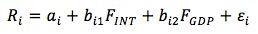### Why should I choose AnalystNotes?

Simply put: AnalystNotes offers the best value and the best product available to help you pass your exams.

##### Subject 2. Factors and Types of Multifactor Models
A factor is a variable or a characteristic with which individual asset returns are correlated. Multifactor models permit a nuanced view of risk that is more granular than the single-factor approach allows.

• They describe the return on an asset in terms of the risk of the asset with respect to a set of factors.
• They generally include systematic factors, which explain the average returns of a large number of risky assets. Such factors represent priced risk-risk for which investors require an additional return for bearing.

According to the type of factors used there are three categories of multifactor models.

Macroeconomic Factor Models

In macroeconomic factor models, the factors are surprises in macroeconomic variables that significantly explain equity returns. Surprise is defined as actual minus forecasted value and has an expected value of zero. Factors such as GDP, interest rates, and inflation can be understood as affecting either the expected future cash flows of companies or the interest rate used to discount these cash flows back to the present.

The key to understanding macroeconomic factor models is that the variables that explain returns are surprises (the unexpected part) because the predicted values have been reflected in asset prices.

Consider a two-factor macroeconomic model (inflation rate and GDP growth):where:

• Ri = the return for asset i
• ai = expected return for asset i in absence of any surprises
• bi1 = Interest rates surprise sensitivity of asset i. This is a slope coefficient which is interpreted as the interest rate factor sensitivity of asset i.
• FINT = surprise in interest rates. This is the interest rates factor surprise.
• bi2 = GDP surprise sensitivity of asset i. This is the GDP factor sensitivity of the asset i.
• FGDP = surprise in the GDP growth. This is the GDP factor surprise, the difference between the expected value and the actual value of the GDP.
• εi = firm-specific surprises (the portion of the return to asset i not explained by the factor model)

This model says that stock returns are explained by surprises in GDP growth and interest rates. The regression analysis is usually used to estimate assets' sensitivities to the factors.

Using the macroeconomic factor model, the expected return for a stock equals the intercept. The expected return on a portfolio of two stocks is thus: E(RP) = wi E(Ri) + wj E(Rj)

Fundamental Factor Models

In fundamental factor models, the factors are attributes of stocks or companies that are important in explaining cross-sectional differences in stock prices. The fundamental factors include book-value-to-price ratio, market cap, P/E ratio, financial leverage, and earnings growth rate.

• In fundamental factor models the factors are stated as returns rather than surprises.

• The factor sensitivities (attributes) are usually specified first in fundamental factor models, and then the factor returns are estimated through regressions. In contrast, in macroeconomic factor models the factor (surprise) series is first developed and then the factor sensitivities are estimated through regressions.

• Macroeconomic factors are usually small in number. Fundamental factors are often large in number, providing a more detailed picture of risk in terms that are easily related to company and security characteristics.

• The intercept in a macroeconomic model is the stock's expected return. The intercept in a fundamental model is the expected return for stocks with factor sensitivities equal to the market-wide averages.

The factors of most fundamental factor models may be classified as company fundamental factors, company share-related factors, or macroeconomic factors.

Statistical Factor Models

In statistical factor models, statistical methods are applied to a set of historical returns to determine portfolios that explain historical returns in one of two senses. In factor analysis models, the factors are the portfolios that best explain (reproduce) historical return covariances. In principal-components models, the factors are portfolios that best explain (reproduce) historical return variances.

APT versus Multifactor Models:

• The APT is a cross-sectional equilibrium model. It explains the variation across assets' expected returns. The multifactor model is a time-series regression. It explains the variation over time in returns for one asset.
• The intercept term in a macroeconomic factor model is the investment's expected return. The intercept in the APT is the risk-free rate.

User Comment
chris54321 I hate studying, all i want to do is eat chips with cheese and watch videos
sebjiguet That's the only smart comment I have seen on this web site :)
chris54321 i prefer pizza. chips with cheese is not healthy
sikwingo Pizza, got cheese too lol. Not healthy either, but better than studying
triplea412 any good study whiskeys?
pmdallman Ha... not maliciously meant - but how did you guys get to level 2 with that attitude ;)
chau76 understand the 2-factor (inflation, GDP) macroeconomic model
thebkr777 Was going to be disciplined and study all day but screw it let's go be fat...
CFAJ pizza really isn't any better than chips with cheese
CFAJ (healthwise)